### Home > CC1 > Chapter 7 > Lesson 7.2.2 > Problem7-56

7-56.
1. Calculate each of the following products without using a calculator. Homework Help ✎1.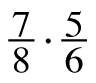2. (3.1)(0.02)

3.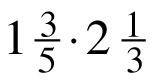4.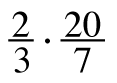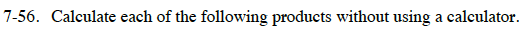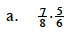$\frac{a}{b}\cdot\frac{c}{d}=\frac{a \cdot\ c}{b \cdot\ d}$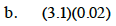Use a generic rectangle, as shown below.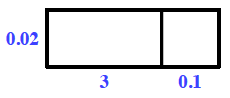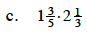Change the mixed numbers into fractions and then refer to part (a) for help.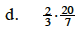$\frac{40}{21}=1\frac{19}{21}$